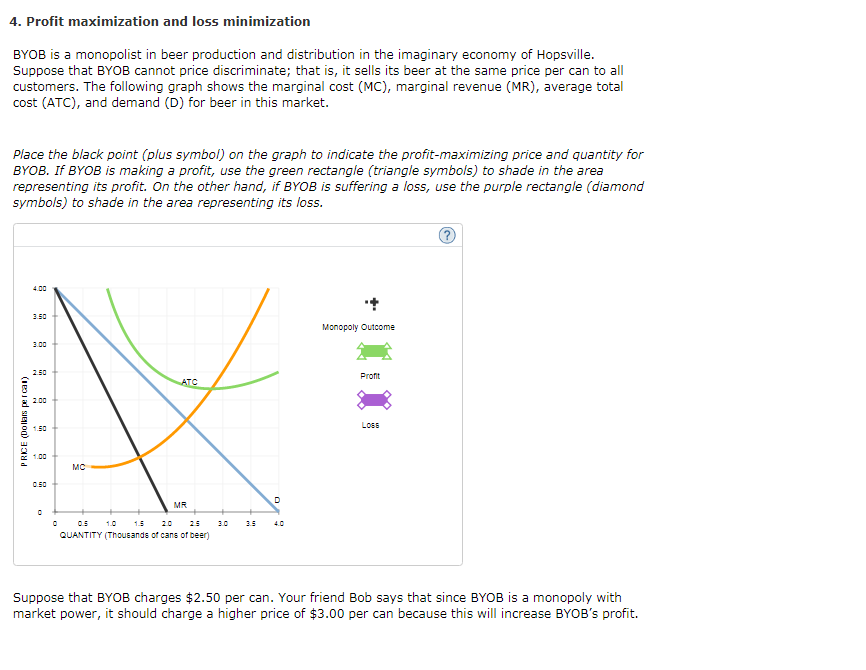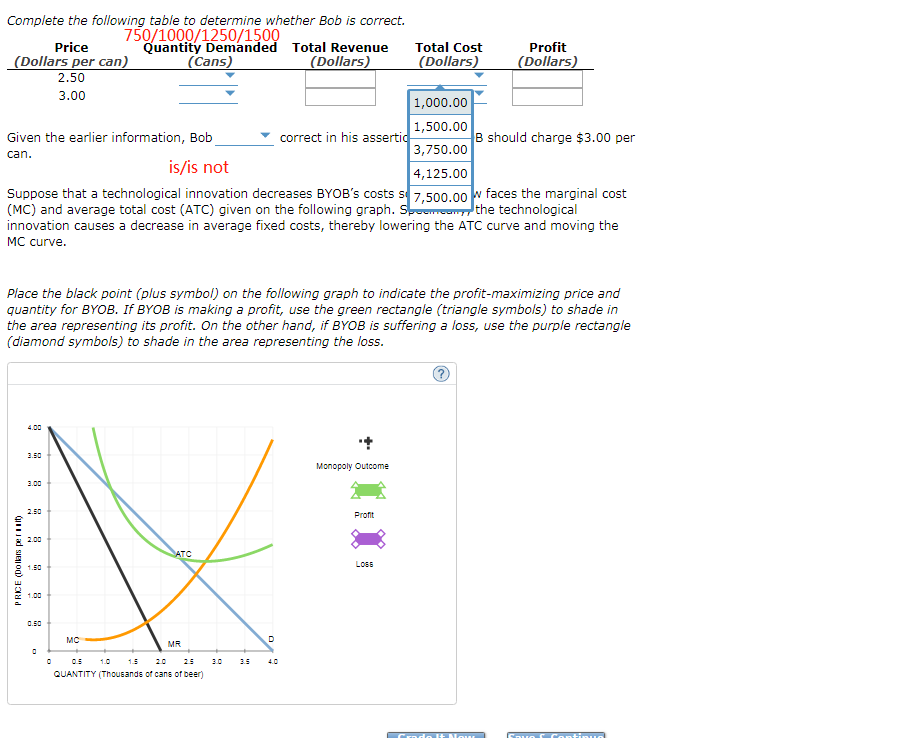### Create an Account

Home / Questions / 4. Profit maximization and loss minimization BYOB is a monopolist in beer production and distributio

# 4. Profit maximization and loss minimization BYOB is a monopolist in beer production and distributio4. Profit maximization and loss minimization BYOB is a monopolist in beer production and distribution in the imaginary economy of Hopsville. Suppose that BYOB cannot price discriminate; that is, it sells its beer at the same price per can to all customers. The following graph shows the marginal cost (MC), marginal revenue (MR), average total cost (ATC), and demand (D) for beer in this market Place the black point (plus symbol) BYOB. If BYOB is making a profit, use the green rectangle (triangle symbols) to shade in the area representing its profit. On the other hand, if BYOB is suffering a loss, symbols) to shade in the area representing its loss. on the graph to indicate the profit-maximizing price and quantity for use the purple rectangle (diamond 4.00 350 Monopoly Outcome 300 250 Profit АTC 200 LOGE 1.50 1.00 мс MR 1.0 15 20 25 30 40 QUANTITY (Thousands of cans of beer) Suppose that BYOB charges \$2.50 per can. Your friend Bob says that since BYOB is a monopoly with market power, it should charge a higher price of \$3.00 per can because this will increase BYOB&#39;s profit. Complete the following table to determine whether Bob is correct. 750/1000/1250/1500 Quantity Demanded Total Revenue Price Total Cost Profit (Dollars per can) (Dollars) (Dollars) (Cans) (Dollars) 2.50 3.00 1,000.00 1,500.00 B should charge \$3.00 per correct in his assertic Given the earlier information, Bob 3,750.00 can. is/is not 4,125.00 Suppose that a technological innovation decreases BYOB&#39;s costs s 7.500,00 (MC) and average total cost (ATC) given on the following graph. S innovation causes a decrease in average fixed costs, thereby lowering the ATC curve and moving the MC curve. faces the marginal cost the technological W Place the black point (plus symbol) on the following graph to indicate the profit-maximizing price and quantity for BYOB. If BYOB is making a profit, use the green rectangle (triangle symbols) to shade in the area representing its profit. On the other hand, if BYOB is suffering a loss, use the purple rectangle (diamond symbols) to shade in the area representing the loss. 4.00 3.50 Monopoly Outcome 3.00 2.50 Profit 2.00 ATC LOSE 1.50 1.00 MC MR. 05 1.0 15 20 25 30 35 40 QUANTITY (Thousands of cans of beer) Couo C Conti uol SIEO) dd

Apr 06 2020 View more View LessSubscribe To Get Solution